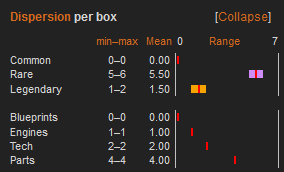## FANDOM

5,230 PagesSOURCE EDITOR ONLY!This page uses LaTeX markup to display mathematical formulas. Editing the page with the VisualEditor or Classic rich-text editor disrupts the layout.Do not even switch to one of these editors while editing the page!For help with mathematical symbols, see Mathematical symbols and expressions.

In mathematics and statistics, deviation is a measure of difference between the observed value of a variable and some other value, often that variable's mean. The sign of the deviation reports the direction of that difference (the deviation is positive when the observed value exceeds the reference value). The magnitude of the value indicates the size of the difference.

## Types

A deviation that is a difference between an observed value and the true value of a quantity of interest (such as a population mean) is an error and a deviation that is the difference between the observed value and an estimate of the true value (such an estimate may be a sample mean) is a residual.

### Unsigned or absolute deviation

In statistics, the absolute deviation of an element of a data set is the absolute difference between that element and a given point. Typically the deviation is reckoned from the central value, being construed as some type of average, most often the median or sometimes the mean of the data set.

$D_i = |x_i-m(X)|$

where

$D_i$ is the absolute deviation,
$x_i$ is the data element, and
$m(X)$ is the chosen measure of central tendency of the data set—sometimes the mean ($\bar{x}$), but most often the median.

## Measures

Statistics of the distribution of deviations are used as measures of statistical dispersion, such as the frequently used standard deviation. It uses squared deviations, and has desirable properties.

## Examples

### Dispersion in Pro Kit BoxesAbsolute deviations (bars) from the means (red lines) in McLaren 600LT Basic Kit Boxes as of July 4, 2019Absolute deviations from the means in Daily Kit Boxes as of July 4, 2019 Absolute deviations from the arithmetic mean are displayed by the Card distribution per box tab in infoboxes of Pro Kit Box pages. The images to the right show examples for the McLaren 600LT and the .

When comparing the means of rare cards in R&D Basic Kit Boxes (5.50) and common cards in Daily Kit Boxes (5.78), it can be seen that despite similar mean values, the deviations are different: While the number of rare cards in R&D Basic Kit Boxes only deviates by ±0.5 cards (5 − 5.5 and 6 − 5.5, respectively), the absolute deviations for common cards in Daily Kit Boxes range from −3.78 to 3.22 cards (2 − 5.78 and 9 − 5.78, respectively). While players can expect a number of rare cards from R&D Basic Kits Boxes that is close to the mean, common cards in Daily Kit Boxes are more dispersed.

If there is no bar at all around the red lines, there are no deviations and no dispersion. This indicates that the number of cards is not random, but fixed.

### Deviations from official drop ratesCommon, rare and legendary cards in Random Boxes during the 2019 Spring Update

Deviations from official drop rates of Pro Kit Boxes can also be a useful indicator. According to the law of large numbers, the average relative frequencies of common, rare and legendary cards will converge to their respective drop rates after a certain number of revealed cards (200 are acceptable in most cases), and almost surely as the number approaches infinity. This can be seen in the chart to the right where the relative frequencies inRandom Boxes are shown together with the corresponding drop rates.

If the real statistical outcomes of common, rare and legendary cards are congruent with the drop rates of a box, other statistics normally are reliable as well, for example the frequencies of Engine, Tech and Part cards for which official drop rates do not exist.Common, rare and legendary cards in Finish Line Boxes during the 2019 Spring Update

The , however, notorious for displaying wrong drop rates, shows a different picture. During the 2019 Spring Update, its frequencies changed direction several times and were mostly far off the official drop rates.

If average frequencies stabilize at other values than the drop rates, it is an indicator that the drop rates are simply wrong. In this case, however, statistics are most probably reliable as the outcomes follow the law of large numbers and converge to expected values—these values are just different from the official (wrong) ones.

If frequencies do not stabilize at all, but keep converging or diverging, players have to use statistical data with caution. This indicates quite surely that the box content has been changed several times without adjusting the drop rates, and players can rely neither on drop rates nor on statistical data, as statistical data always has a certain time lag until the sample size is large enough to reflect changes.